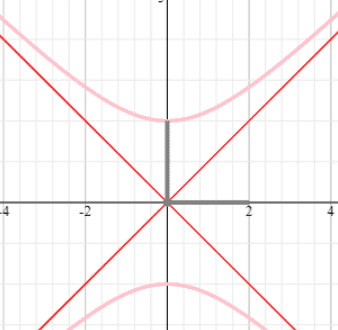# Graph the Cartesian equation

Fatima Hasan

## Homework Statement

Identify the particle's path by finding a Cartesian equation for it . Graph the Cartesian equation . indicate the portion of the graph traced by the particle .
##x=2sinh t## , ##y=2cosh t## , ##-\infty < t < \infty##

## Homework Equations

##cosh^2 t - sinh^2 t = 1##

## The Attempt at a Solution

##sinh t = \frac{x}{2}##
Square both sides :
##sinh^2 t = \frac{x^2}{4}## (1)
##cosh t = \frac{y}{2}##
Square both sides :
##cosh^2 t = \frac{y^2}{4}## (2)
(2) - (1) :
##cosh^2 t - sinh^2 t = 1##
##\frac{y^2}{4} - \frac{x^2}{4} = 1##
put x= 0 → y=±2#### Attachments

Last edited:

Staff Emeritus
Gold Member

What do you know about the possible values of ##\cosh t## for ##-\infty < t < \infty##?

Fatima Hasan
What do you know about the possible values of ##\cosh t## for ##-\infty < t < \infty##?
The Cartesian equation forms a parbola opening up . Right ?

Staff Emeritus
Gold Member
I just noticed something else.
$$\frac{x^2}{4} - \frac{y^2}{4} =1$$
is not correct, but I think this is a "typo".

Mentor
The Cartesian equation forms a parbola opening up . Right ?
No. The graph you show is not a parabola.

Mentor
Identify the particle's path by finding a Cartesian equation for it .
Graph the Cartesian equation . indicate the portion of the graph traced by the particle .
##x=2sinh t## , ##y=2cosh2## , ##-\infty < t < \infty##
Should the second equation be ##y = 2\cosh(t)##?

Fatima Hasan
Should the second equation be ##y = 2\cosh(t)##?
yeah

Mentor
I agree with @George Jones's comment in post #4.
(2) - (1) :
##cosh^2 t - sinh^2 t = 1##
##\frac{x^2}{4} - \frac{y^2}{4} = 1##
Take a closer look at the work I've quoted above.

Fatima Hasan
##\frac{y^2}{4} - \frac{x^2}{4}## forms a hyperbola and to graph it , we should go 2 units up from center point .
##cosh ( t) ## for ##-\infty < t < \infty## is always positive .

Fatima Hasan
I agree with @George Jones's comment in post #4.
Take a closer look at the work I've quoted above.
##\frac{y^2}{4} - \frac{x^2}{4} = 1##

Mentor
##\frac{y^2}{4} - \frac{x^2}{4} = 1##
Yes, that looks better.

Staff Emeritus
Gold Member
##\frac{y^2}{4} - \frac{x^2}{4} = 1## forms a hyperbola

Yes, a big hint is given by the names of the functions in the original equations.•Fatima Hasan
Mentor
View attachment 231720
Is it correct ?
No.
The equation ##\frac{y^2}{4} - \frac{x^2}{4} = 1## is NOT a parabola. The graph you showed appears to be the graph of ##y = x^2 + 2##, which is completely unrelated to your equation.

Mentor
Bingo!
(Meaning, yes, that's it.)

•Fatima Hasan
Fatima Hasan
Bingo!
(Meaning, yes, that's it.)